Scroll

# Programming Col Sig (means): Classical Student Test (with and without estimator)

Follow

This article demonstrates how to script the formula for Student Test and Student Test using estimator for testing means. Formula and syntax are shown for tables using regular mean calculations as well as Script (mean) calculations which would be used in a mean summary table.

The .qes file and portfolio containing the examples discussed is attached: Table Arithmetic Examples 15.rar.

In this article we will look at programming column significance tests for means.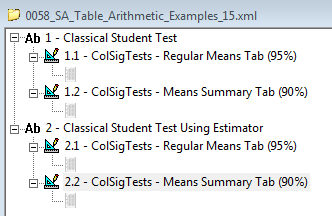For each test I will show how to set up for:

• a table that shows regular means - i.e. ‘Mean’ calculation at the bottom of a scaled rating question in the rows. (A)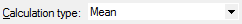See tab definitions: ColSigTests - Regular Means Tab (95%)

• a mean summary table - i.e. ‘Script (mean)’ calculation which references a scaled question to be filtered by the rows and columns of the tab definition. (B)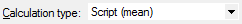See tab definitions: ColSigTests - Means Summary Tab (90%)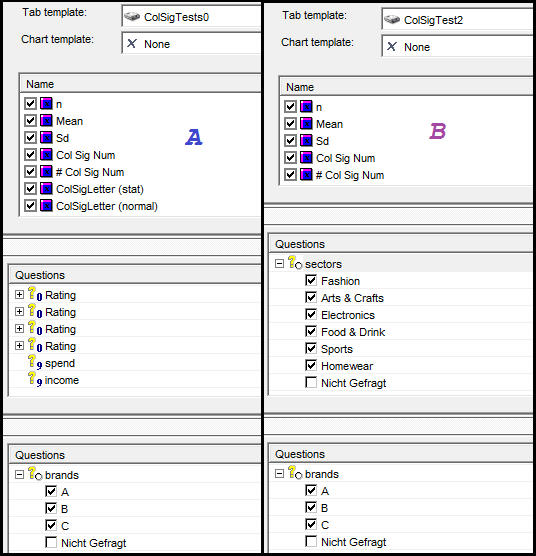Classical Student Test

Let’s refresh ourselves on the formula for this: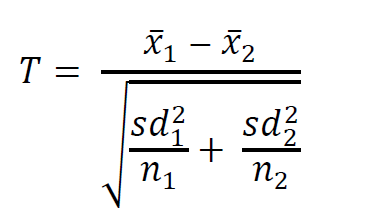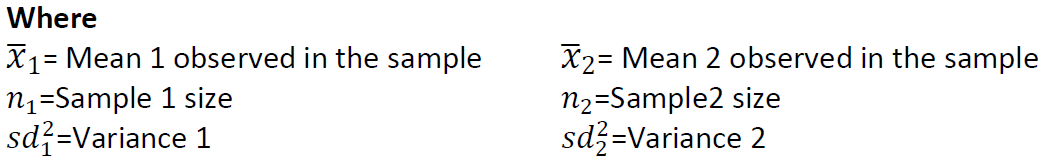Since the formula references the standard deviation, we’ll need to add a calculation for this as well.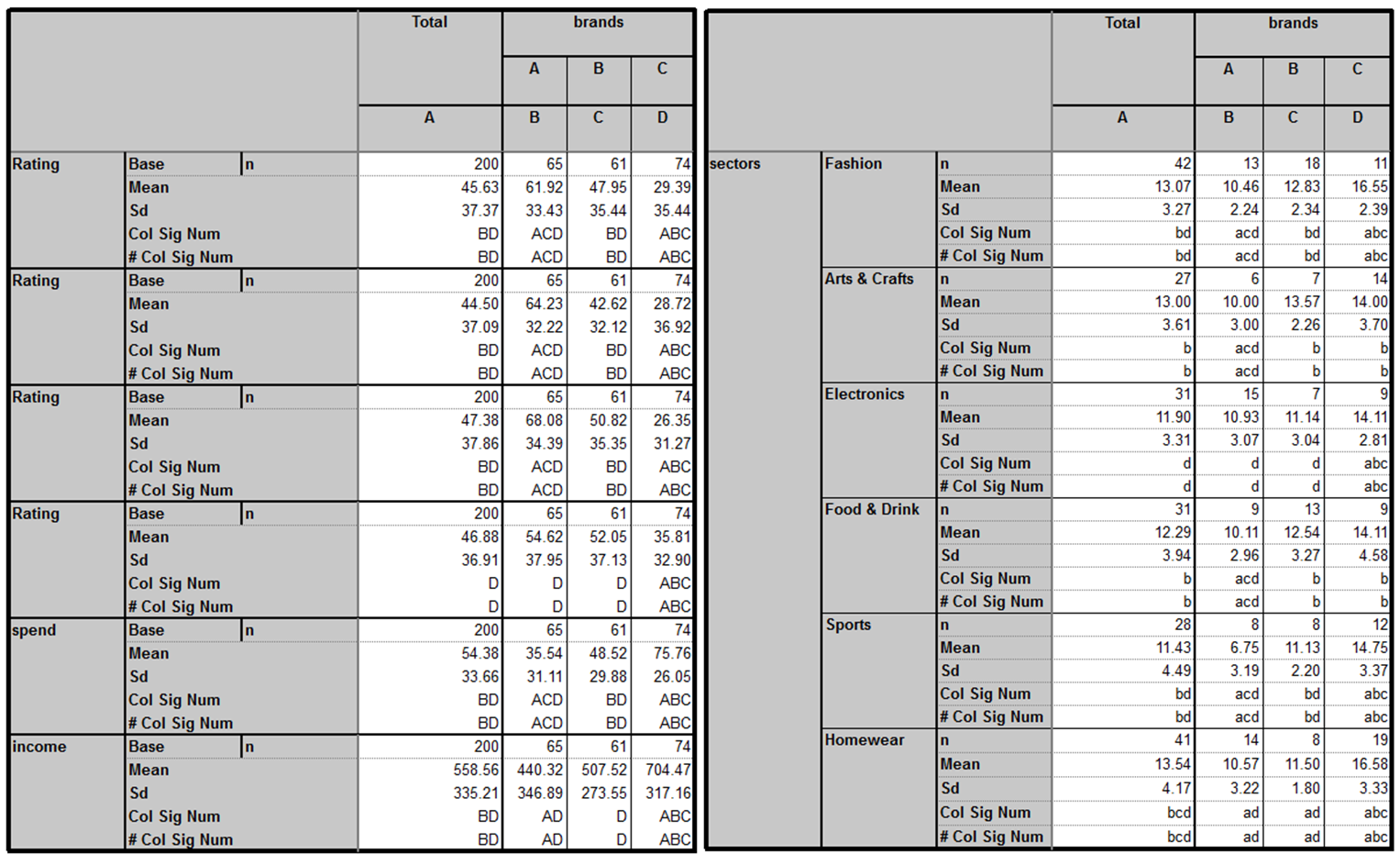Then in (# Col Sig Num) we add the script: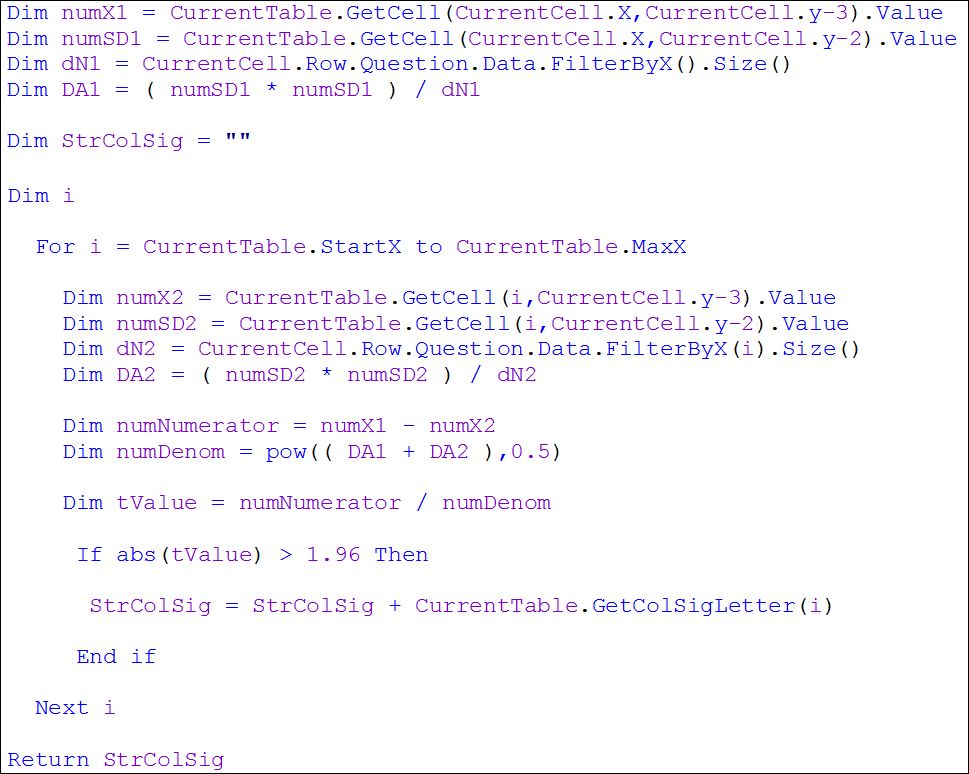The points to note here are: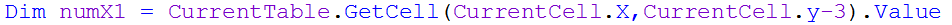This grabs the value from the calculation 3 cells above the current calculation’s cell  -  Mean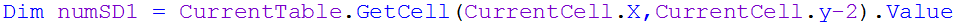This grabs the value from the calculation 2 cells above the current calculation’s cell  -  Sd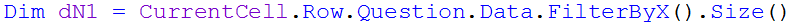This grabs the base – we could use the previous syntax replacing the Y co-ordinate reference with: CurrentCell.y-4 but it’s preferable to use ‘FilterBy’ keyword as it’s more dynamic (doesn’t require update if calculations added or moved).

You’ll note that we have defined numX1, numSD1 and dN1 all outside of a loop and then defined their counterparts numX2, numSD2 and dN2 inside of a loop (iterates through each data column). So each column (1) is tested against all other columns (2) in turn.

For the mean summary table we need to add: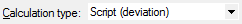You’ll note for the script in this tab definition we use: (If abs(tValue) > 1.645 Then)  the instead of:  (If abs(tValue) > 1.96 Then). That’s because this test is done at the 90%  confidence level rather than 95%.

Classical Student Test (using estimator)

The formula for this: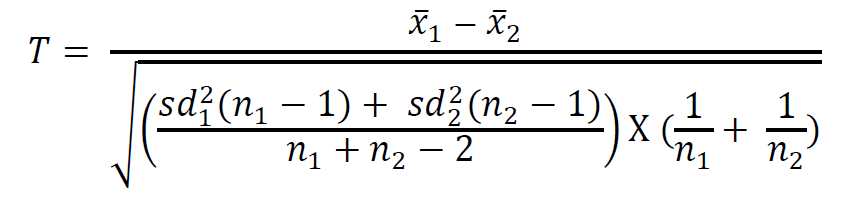The tables look like this: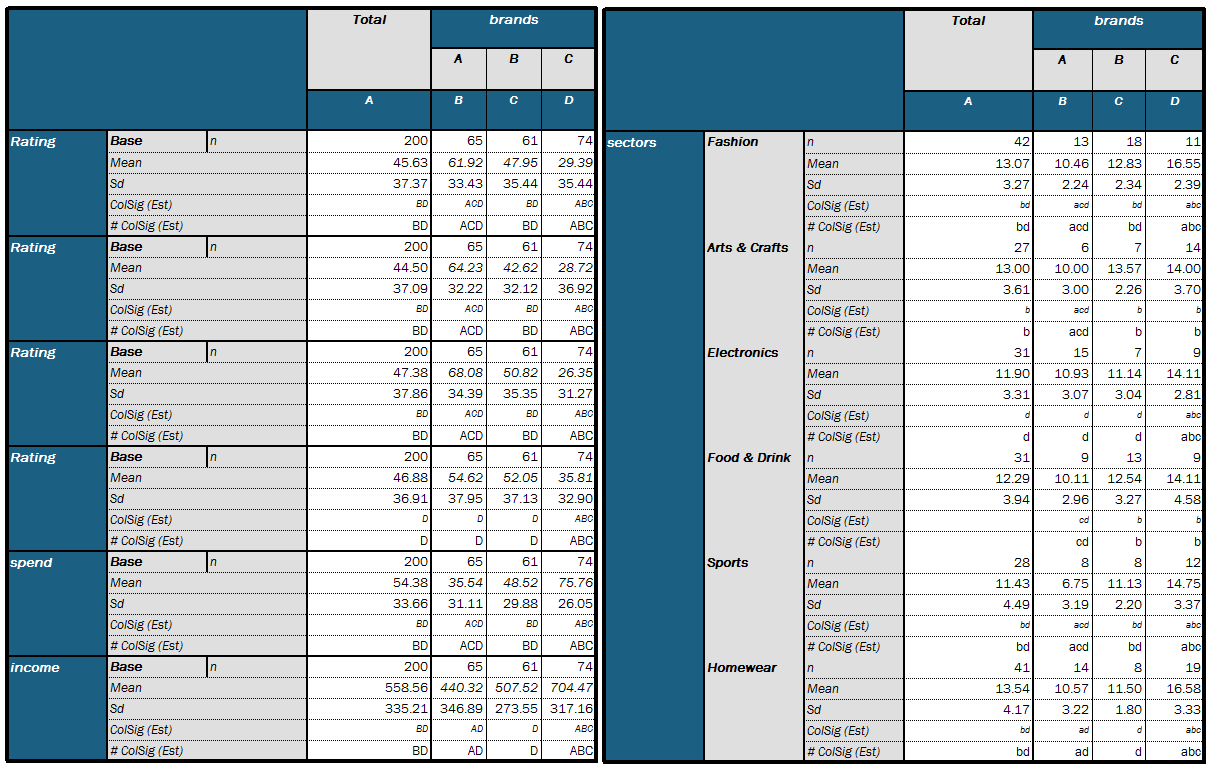And the script in # ColSig (Est):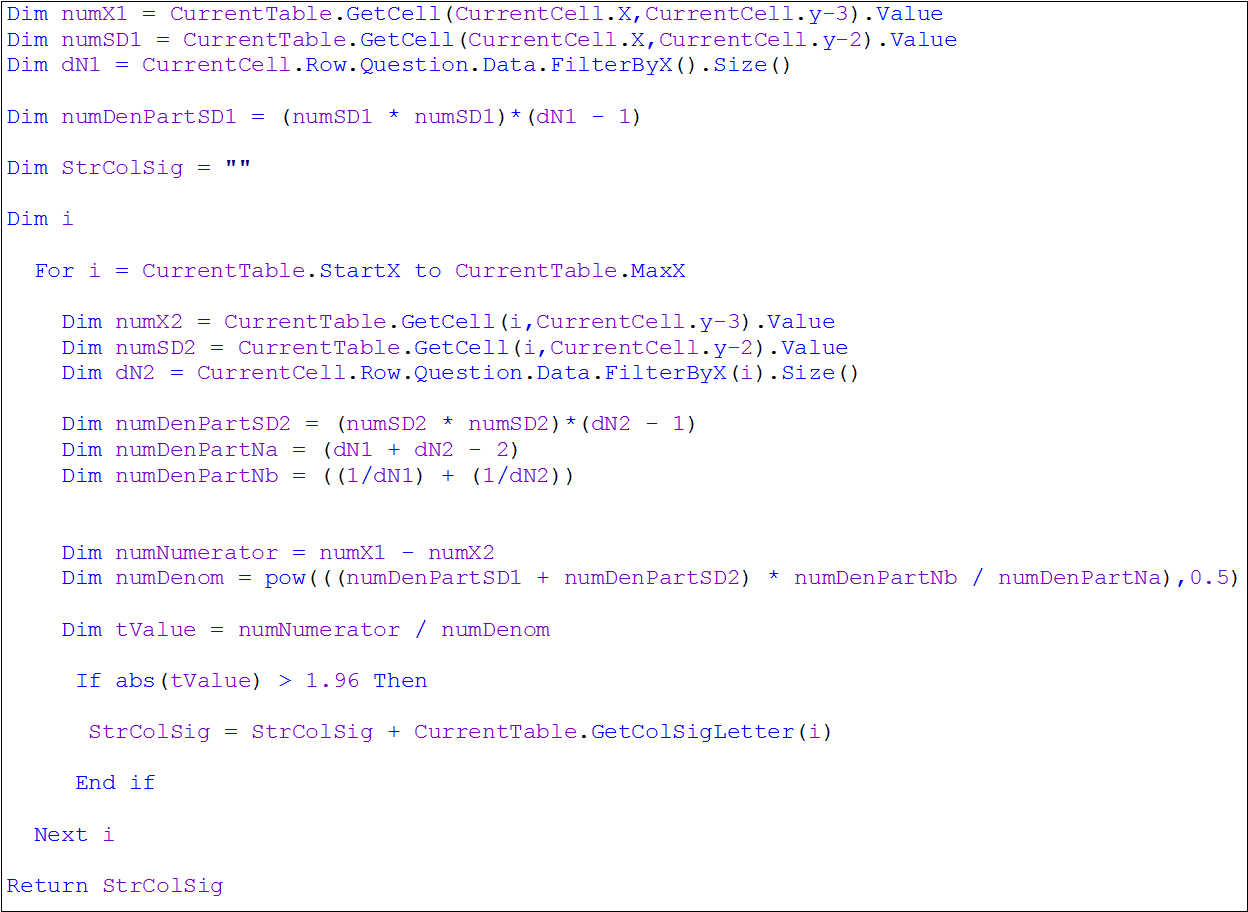The article is also attached as a word document: Programming Col Sig for Means.docx